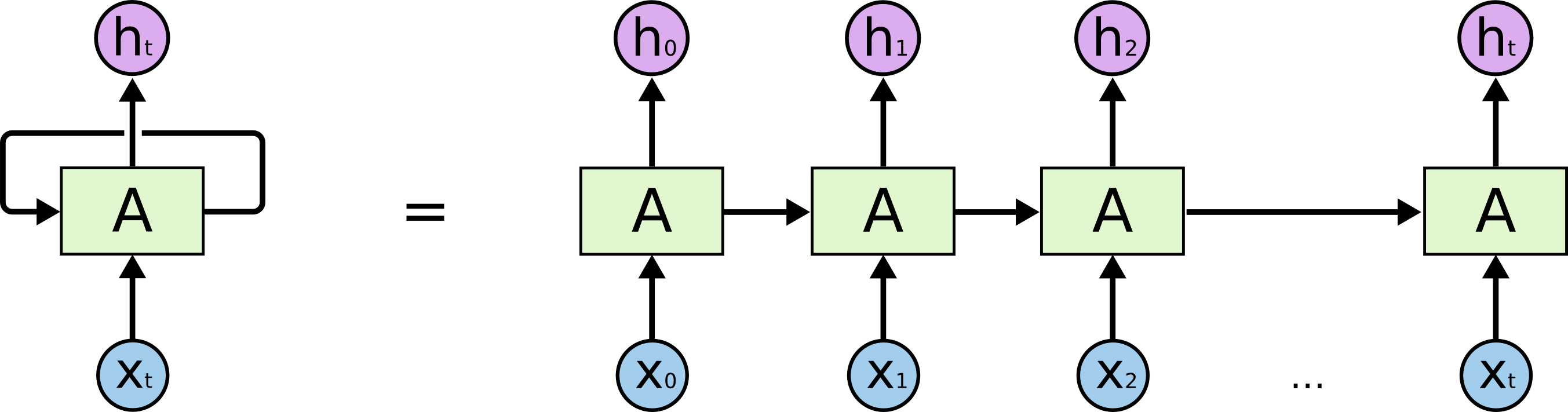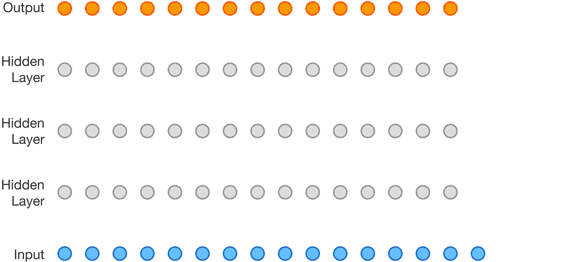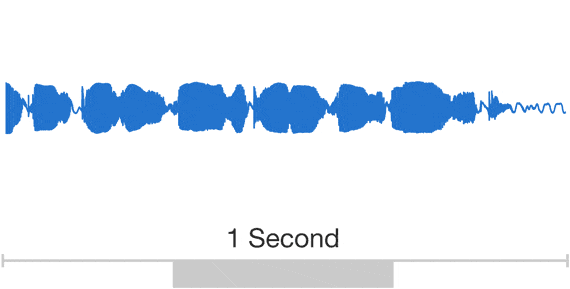My current project involves working with deep autoregressive models: a class of remarkable neural networks that aren’t usually seen on a first pass through deep learning. These notes are a quick write-up of my reading and research: I assume basic familiarity with deep learning, and aim to highlight general trends and similarities across autoregressive models, instead of commenting on individual architectures.

tldr: Deep autoregressive models are sequence models, yet feed-forward (i.e. not recurrent); generative models, yet supervised. They are a compelling alternative to RNNs for sequential data, and GANs for generation tasks.

## Deep Autoregressive Models

To be explicit (at the expense of redundancy), this blog post is about deep autoregressive generative sequence models. That’s quite a mouthful of jargon (and two of those words are actually unnecessary), so let’s unpack that.

1. Deep
• Well, these papers are using TensorFlow or PyTorch… so they must be “deep”• You would think this word is unnecessary, but it’s actually not! Autoregressive linear models like ARMA or ARCH have been used in statistics, econometrics and financial modelling for ages.
2. Autoregressive
• Stanford has a good introduction to autoregressive models, but I think a good way to explain these models is to compare them to recurrent neural networks (RNNs), which are far more well-known.Obligatory RNN diagram. Source: Chris Olah.
• Like an RNN, an autoregressive model’s output $$h_t$$ at time $$t$$ depends on not just $$x_t$$, but also $$x$$’s from previous time steps. However, unlike an RNN, the previous $$x$$’s are not provided via some hidden state: they are given as just another input to the model.
• The following animation of Google DeepMind’s WaveNet illustrates this well: the $$t$$th output is generated in a feed-forward fashion from several input $$x$$ values.1WaveNet animation. Source: Google DeepMind.
• Put simply, an autoregressive model is merely a feed-forward model which predicts future values from past values.
• I’ll explain this more later, but it’s worth saying now: autoregressive models offer a compelling bargain. You can have stable, parallel and easy-to-optimize training, faster inference computations, and completely do away with the fickleness of truncated backpropagation through time, if you are willing to accept a model that (by design) cannot have infinite memory. There is recent research to suggest that this is a worthwhile tradeoff.
3. Generative
• Informally, a generative model is one that can generate new data after learning from the dataset.
• More formally, a generative model models the joint distribution $$P(X, Y)$$ of the observation $$X$$ and the target $$Y$$. Contrast this to a discriminative model that models the conditional distribution $$P(Y|X)$$.
• GANs and VAEs are two families of popular generative models.
• This is unnecessary word #1: any autoregressive model can be run sequentially to generate a new sequence! Start with your seed $$x_1, x_2, ..., x_k$$ and predict $$x_{k+1}$$. Then use $$x_2, x_3, ..., x_{k+1}$$ to predict $$x_{k+2}$$, and so on.
4. Sequence model
• Fairly self explanatory: a model that deals with sequential data, whether it is mapping sequences to scalars (e.g. language models), or mapping sequences to sequences (e.g. machine translation models).
• Although sequence models are designed for sequential data (duh), there has been success at applying them to non-sequential data. For example, PixelCNN (discussed below) can generate entire images, even though images are not sequential in nature: the model generates a pixel at a time, in sequence!2
• Notice that an autoregressive model must be a sequence model, so it’s redundant to further describe these models as sequential (which makes this unnecessary word #2).

A good distinction is that “generative” and “sequential” describe what these models do, or what kind of data they deal with. “Autoregressive” describes how these models do what they do: i.e. they describe properties of the network or its architecture.

## Some Architectures and Applications

Deep autoregressive models have seen a good degree of success: below is a list of some of examples. Each architecture merits exposition and discussion, but unfortunately there isn’t enough space here to devote to do any of them justice.

These models have also found applications: for example, Google DeepMind’s ByteNet can perform neural machine translation (in linear time!) and Google DeepMind’s Video Pixel Network can model video.3

## Some Thoughts and Observations

1. Given previous values $$x_1, x_2, ..., x_t$$, these models do not output a value for $$x_{t+1}$$, they output the predictive probability distribution $$P(x_{t+1} | x_1, x_2, ..., x_t)$$ for $$x_{t+1}$$.
• If the $$x$$’s are discrete, then you can do this by outputting an $$N$$-way softmaxxed tensor, where $$N$$ is the number of discrete classes. This is what the original PixelCNN did, but gets problematic when $$N$$ is large (e.g. in the case of WaveNet, where $$N = 2^{16}$$).
• If the $$x$$’s are continuous, you can model the probability distribution itself as the sum of basis functions, and having the model output the parameters of these basis functions. This massively reduces the memory footprint of the model, and was an important contribution of PixelCNN++.
• Theoretically you could have an autoregressive model that doesn’t model the conditional distribution… but most recent models do.
2. Autoregressive models are supervised.
• With the success and hype of GANs and VAEs, it is easy to assume that all generative models are unsupervised: this is not true!
• This means that that training is stable and highly parallelizable, that it is straightfoward to tune hyperparameters, and that inference is computationally inexpensive. We can also break out all the good stuff from ML-101: train-valid-test splits, cross validation, loss metrics, etc. These are all things that we lose when we resort to e.g. GANs.
3. Autoregressive models work on both continuous and discrete data.
• Autoregressive sequential models have worked for audio (WaveNet), images (PixelCNN++) and text (Transformer): these models are very flexible in the kind of data that they can model.
• Contrast this to GANs, which (as far as I’m aware) cannot model discrete data.
4. Autoregressive models are very amenable to conditioning.
• There are many options for conditioning! You can condition on both discrete and continuous variables; you can condition at multiple time scales; you can even condition on latent embeddings or the outputs of other neural networks.
• There is one ostensible problem with using autoregressive models as generative models: you can only condition on your data’s labels. I.e. unlike a GAN, you cannot condition on random noise and expect the model to shape the noise space into a semantically (stylistically) meaningful latent space.
• Google DeepMind followed up their original PixelRNN paper with another paper that describes one way to overcome this problem. Briefly: to condition, they incorporate the latent vector into the PixelCNN’s activation functions; to produce/learn the latent vectors, they use a convolutional encoder; and to generate an image given a latent vector, they replace the traditional deconvolutional decoder with a conditional PixelCNN.
• WaveNet goes even futher and employs “global” and “local” conditioning (both are achieved by incorporating the latent vectors into WaveNet’s activation functions). The authors devise a battery of conditioning schemes to capture speaker identity, linguistic features of input text, music genre, musical instrument, etc.
5. Generating output sequences of variable length is not straightforward.
• Neither WaveNet nor PixelCNN needed to worry about a variable output length: both audio and images are comprised of a fixed number of outputs (i.e. audio is just $$N$$ samples, and images are just $$N^2$$ pixels).
• Text, on the other hand, is different: sentences can be of variable length. One would think that this is a nail in a coffin, but thankfully text is discrete: the standard trick is to have a “stop token” that indicates that the sentence is finished (i.e. model a full stop as its own token).
• As far as I am aware, there is no prior literature on having both problems: a variable-length output of continuous values.
6. Autoregressive models can model multiple time scales
• In the case of music, there are important patterns to model at multiple time scales: individual musical notes drive correlations between audio samples at the millisecond scale, and music exhibits rhythmic patterns over the course of minutes. This is well illustrated by the following animation:Audio exhibits patterns at multiple time scales. Source: Google DeepMind.
• There are two main ways model many patterns at many different time scales: either make the receptive field of your model extremely wide (e.g. through dilated convolutions), or condition your model on a subsampled version of your generated output, which is in turn produced by an unconditioned model.
• Google DeepMind composes an unconditional PixelRNN with one or more conditional PixelRNNs to form a so-called “multi-scale” PixelRNN: the first PixelRNN generates a lower-resolution image that conditions the subsequent PixelRNNs.
• WaveNet employs a different technique and calls them “context stacks”.
7. How the hell can any of this stuff work?
• RNNs are theoretically more expressive and powerful than autoregressive models. However, recent work suggests that such infinite-horizon memory is seldom achieved in practice.
• To quote John Miller at the Berkeley AI Research lab:

Recurrent models trained in practice are effectively feed-forward. This could happen either because truncated backpropagation through time cannot learn patterns significantly longer than $$k$$ steps, or, more provocatively, because models trainable by gradient descent cannot have long-term memory.

1. There’s actually a lot more nuance than meets the eye in this animation, but all I’m trying to illustrate is the feed-forward nature of autoregressive models.

2. I personally think it’s breathtakingly that machines can do this. Imagine your phone keyboard’s word suggestions (those are autoregressive!) spitting out an entire novel. Or imagine weaving a sweater but you had to choose the color of every stitch, in order, in advance.

3. In case you haven’t noticed, Google DeepMind seemed to have had an infatuation with autoregressive models back in 2016.

Tags:

Updated:

## Want to hear more from me?

Subscribe to my newsletter! My thoughts on what I'm reading and learning, delivered once a month.
More information here. Newsletter archive here.